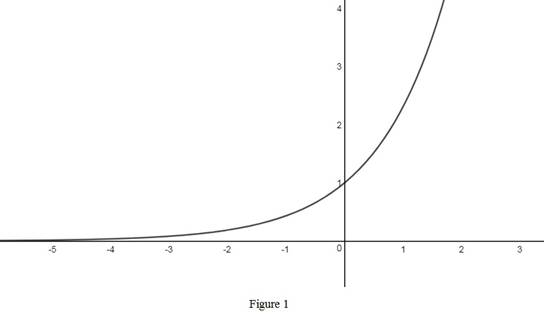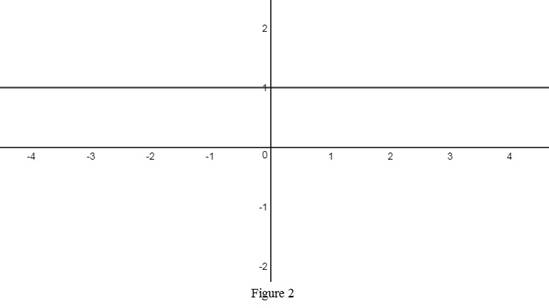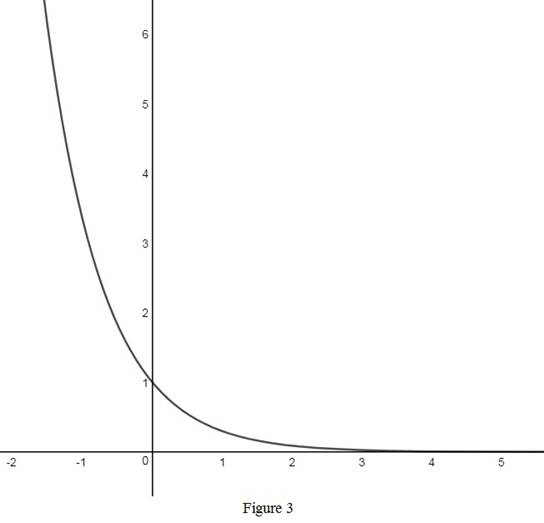# An equation of the exponential function with base b &gt; 0 .### Single Variable Calculus: Concepts...

4th Edition
James Stewart
Publisher: Cengage Learning
ISBN: 9781337687805### Single Variable Calculus: Concepts...

4th Edition
James Stewart
Publisher: Cengage Learning
ISBN: 9781337687805

#### Solutions

Chapter 1.5, Problem 5E

(a)

To determine

## To write: An equation of the exponential function with base b>0.

Expert Solution

The equation that defines the exponential function is y=bx.

### Explanation of Solution

An exponential function is a function of the form f(x)=ax, where a is a positive constant called its base.

The equation of the exponential function with base b>0 and b1 is y=bx.

Notice that this function is not defined when b<0.

Therefore, the equation of the exponential function with base b>0 is y=bx.

(b)

To determine

### The domain of the exponential function obtained in part (a).

Expert Solution

The domain of the exponential function y=bx is (,).

### Explanation of Solution

The domain of a function is defined as the set of all possible values of the independent variable of the function for which the function is defined.

Consider the exponential function y=bx, whose independent variable is x.

Since the function y=bx is defined for all values of x, its domain will be the set all real values, that is (,).

Therefore, the domain of the exponential function y=bx is (,).

(c)

To determine

### The range of the function y=bx if b≠1.

Expert Solution

The range of the function y=bx if b1 is (0,).

### Explanation of Solution

The range of a function is defined as the set of all possible values of the dependent variable of the function.

Consider the exponential function y=bx, whose dependent variable is y.

Since b>0 and b1, the value of y is always positive.

So the range of the function is the set of all positive real values.

Therefore, the range of the function y=bx if b1 is (0,).

(d)

(i)

To determine

Expert Solution

### Explanation of Solution

The graph of the exponential function y=bx,b>1 is shown below in Figure 1.From Figure 1, it is observed that the graph is monotonically increasing.

(ii)

To determine

Expert Solution

### Explanation of Solution

The graph of the exponential function y=bx,b=1 is shown below in Figure 2.From Figure 2, it is observed that the graph is parallel to x-axis as the function is a constant.

(iii)

To determine

Expert Solution

### Explanation of Solution

The graph of the exponential function y=bx,0<b<1 is shown below in Figure 3.From Figure 3, it is observed that the graph is monotonically decreasing.

### Have a homework question?

Subscribe to bartleby learn! Ask subject matter experts 30 homework questions each month. Plus, you’ll have access to millions of step-by-step textbook answers!